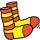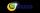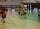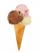# Volleyball

8 girls wants to play volleyball against boys. On the field at one time can be six players per team. How many initial teams of this girls may trainer to choose?

Result

n =  28

#### Solution:Leave us a comment of example and its solution (i.e. if it is still somewhat unclear...):Be the first to comment!#### To solve this verbal math problem are needed these knowledge from mathematics:

Would you like to compute count of combinations?

## Next similar examples:

1. Class pairsIn a class of 34 students, including 14 boys and 20 girls. How many couples (heterosexual, boy-girl) we can create? By what formula?
2. ChordsHow many 4-tones chords (chord = at the same time sounding different tones) is possible to play within 7 tones?
3. TeamsHow many ways can divide 16 players into two teams of 8 member?
4. TrinityHow many different triads can be selected from the group 43 students?
5. BlocksThere are 9 interactive basic building blocks of an organization. How many two-blocks combinations are there?
6. Calculation of CNCalculate: ?
7. Fish tankA fish tank at a pet store has 8 zebra fish. In how many different ways can George choose 2 zebra fish to buy?
8. ConfectioneryThe village markets have 5 kinds of sweets, one weighs 31 grams. How many different ways a customer can buy 1.519 kg sweets.
9. Theorem proveWe want to prove the sentence: If the natural number n is divisible by six, then n is divisible by three. From what assumption we started?
10. ExaminationThe class is 21 students. How many ways can choose two to examination?
11. MATESIn MATES (Small Television tipping) from 35 randomly numbers drawn 5 winning numbers. How many possible combinations there is?
12. TournamentDetermine how many ways can be chosen two representatives from 34 students to school tournament.
13. Ice creamAnnie likes much ice cream. In the shop are six kinds of ice cream. In how many ways she can buy ice cream to three scoop if each have a different flavor mound and the order of scoops doesn't matter?
14. TreesA certain species of tree grows an average of 0.5 cm per week. Write an equation for the sequence that represents the weekly height of this tree in centimeters if the measurements begin when the tree is 200 centimeters tall.
15. PowersExpress the expression ? as the n-th power of the base 10.
16. Nineteenth memberFind the nineteenth member of the arithmetic sequence: a1=33 d=5 find a19
17. Count of trianglesGiven a square ABCD and on each side 8 internal points. Determine the number of triangles with vertices at these points.